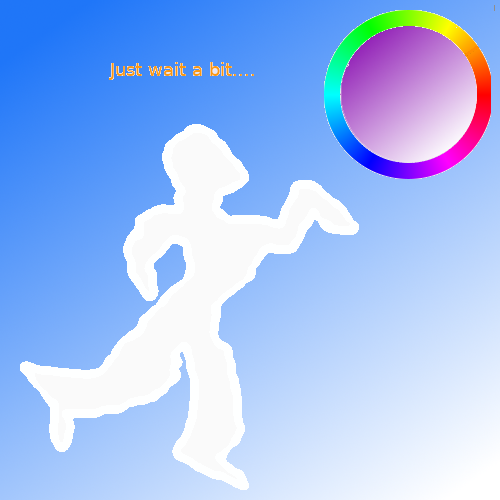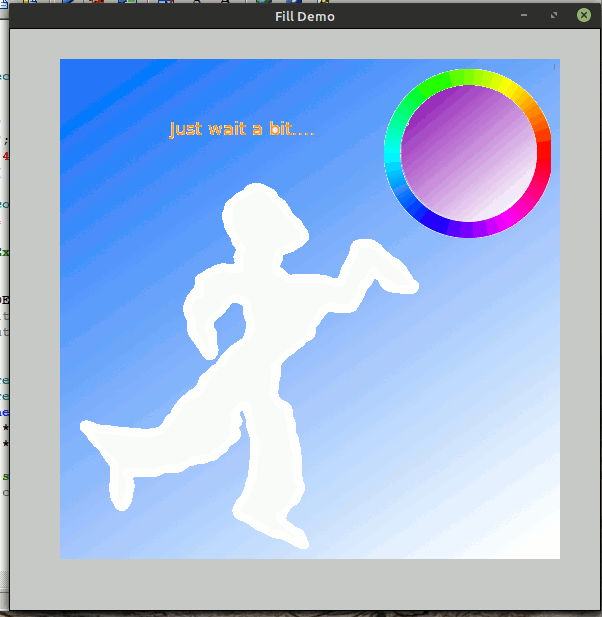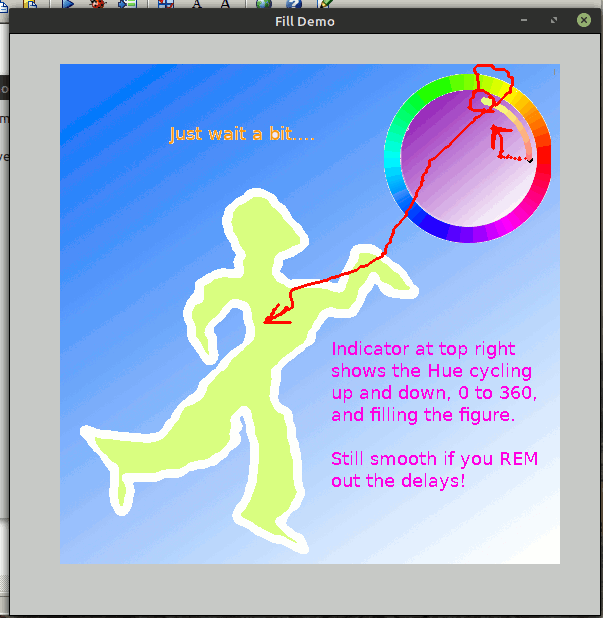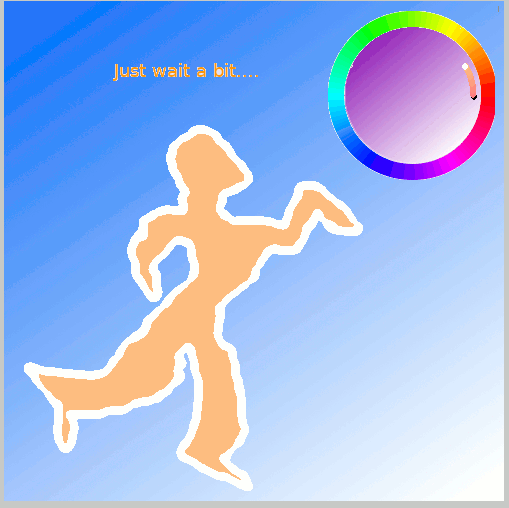# Colour Flood Filling in HSV ( or RGB).-Rapid flood-filling with sequences of H ( hue- the colour); saturation ( fraction of the full colour to use; and V ( value- how far the rest of the RGB triple is between black and white). There's an excellent description of HSV on Wikipedia, from which part of one diagram is used above.

After a delay for you to take in the starting image, part of the right-hand image is filled with a continuous cycle forward and backward through the whole spectral colour range. An indicator rotates showing which H value is currently selected.

You can also alter the S and V values.

If you instead choose RGB description for colour, you can fade white up to grey, or fade between saturated or unsaturated colours.-## Code. Save in a LB directory and save there also from this page, above, the initial 'dancer5.bmp' image.

```    nomainwin

global pi,       col\$, rd, gn, bu

WindowWidth     = 600
WindowHeight    = 610

open "Fill Demo" for graphics_nsb as #w

hw      =hwnd( #w)
calldll #user32, "GetDC", hw as ulong, hdc as ulong

#w   "trapclose quit"
#w   "fill 200 200 200"

x   =250 : y =300
i   =0

#w "drawbmp scr 50 30 ; flush ; down ; size 6"

call delay 3000

xS =520: yS =126: direction =1:i =0

do
#w "color "; rd; " "; gn; " "; bu
#w "set "; xS; " "; yS
call hsv2rgb i, 0.49, 0.99
'call hsv2rgb int( 360 *i /200), 0.99, 0.99         '   hue 0-359, saturation 0-0.99, value 0-0.99
'   alters col\$, rd, gn, bu values
#w "backcolor "; col\$                               '   this is colour to fill WITH
targetcolor   =254 +255 *2^8 +255 *2^16             '   bu *2^16    +gn *2^8 this is colour to fill out TO ie desired border

calldll #gdi32, "ExtFloodFill", hdc as ulong,_
x                  as  long,  y as  long,_
targetcolor        as  long,_
_FLOODFILLBORDER   as  long, result as  long    '   ie fill out 'til this colour is met..
'   replace with _FLOODFILLSURFACE   as  long, result as  long
'   ie fill out 'WHILE this colour is encountered.

scan
if i =359 then direction =0': #w "up; goto 460 126 ; backcolor "; int(256 *rnd( 1)); " "; int(256 *rnd( 1)); " "; int(256 *rnd( 1)); " down ; circlefilled 40"
if i =  1 then direction =1': #w "up; goto 460 126 ; backcolor "; int(256 *rnd( 1)); " "; int(256 *rnd( 1)); " "; int(256 *rnd( 1)); " down ; circlefilled 40"
if direction =0 then i =i -1 else i =i +1
xS  =int( 460 +60 *sin( ( i +90) *3.14159265 /180))
yS  =int( 126 +60 *cos( ( i +90) *3.14159265 /180))
call delay 40
#w "color white ; set "; xS; " "; yS
'#w "backcolor "; col\$
call delay 40
loop until 1 =2

wait
end

sub quit h\$
calldll #user32, "ReleaseDC", hw as ulong, hdc as ulong, ret as void   'release the DCclose #h\$
close #w
end
end sub

sub hsv2rgb h, s, v '                       hue 0-360, saturation 0-1, value 0-1
c   =v *s       '                           chroma
h   =h
x   =c *( 1 -abs( ( ( h /60) mod 2) -1))
m   =v -c   '                               matching adjustment

select case
case h < 60
r = c: g = x: b = 0
case h <120
r = x: g = c: b = 0
case h <180
r = 0: g = c: b = x
case h <240
r = 0: g = x: b = c
case h <300
r = x: g = 0: b = c
case else
r = c: g = 0: b = x
end select

rd      = abs( int( 256 *( r + m)))
gn      = abs( int( 256 *( g + m)))
bu      = abs( int( 256 *( b + m)))
col\$    =right\$( "  " +str\$( rd), 3) +" " +right\$( "   " +str\$( gn), 3) +" " +right\$( "   " +str\$( bu), 3)
end sub